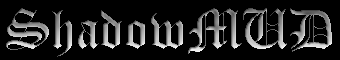Help • Player Commands • Calc

```Syntax: calc [formula]>

A calculator that lets you do integer or floating point calculations.

You may specify a floating point (real) number by ensuring you write the decimal point followed by at least one digit. Generally functions and operators return the same type argument as they are passed; if arguments are mixed real numbers are returned.

Operators:   +, -, *, /, %, ^
Functions:
abs(a)                    - Absolute value (if complex number answer will be float)
arg(a)                    - Argument of a complex number. Returns float.
complex(a, b)             - Returns a+bi
cos(radians)              - returns cosine of angle
div(a, b)                 - integer division ( a/b does floating point)
exp(x)                    - returns e^x
float(a)                  - Converts a to a float with to_float efun
int(a)                    - Rounds a to nearest integer
ln(x)                     - returns natural logarithm of x
polar(abs, arg)           - Returns complex number given polar coordinates
sin(radians)              - returns sine of angle
sqrt(positive_int)        - square root of positive integer as a float
tan(radians)              - returns tangent of angle
to_int(a)                 - Converts a to an integer using to_int efun

Constants:
e   = 2.718282            i   = 0+1i                pi  = 3.141593

Example: calc (2+-1)*-sin(1)
calc polar(abs(3+2i), arg(3+2i))```

Help • Player Commands • Calc